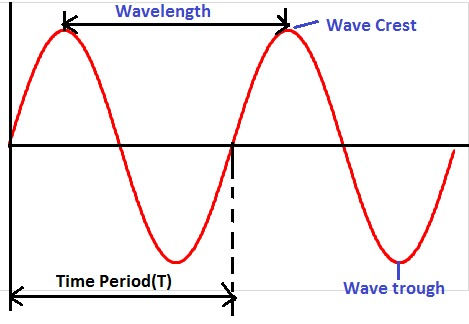Search

# Frequency

The oscillatory signal starts from zero attain the maximum peak attain the maximum peak value crossing the initial position and again come to its initial position.Thus, the number of oscillation completed in one unit time is called the frequency. The frequency term is widely used in oscillatory,rotary and wave motion.

The number of repeating events in a definite time period is called frequency, The SI unit of frequency is Hz. The frequency is also denoted by cycles/second. The frequency of 2 Hz, means the wave completes 2 cycles in one seconds.

Example:

A rotor of an induction motor rotates 1000 rounds in one minute. What is the frequency of rotation.

No of cycles = 1000

Time (t) = 60 Minutes = 60 X60 =3600 Seconds

Frequency = No. of Cycles/Time

= 1000/3600

= 0.27 Hz

The various terms of periodic waveform can be understood with the following diagram.The time period (T) is the time taken to complete one complete cycle. The time period(T) is inversely proportional to frequency. The more the frequency, the lesser is the time period.If frequency of the alternating cycle is 50 Hz. The time period of the waveform is;## Relationship between wavelength and frequency

The distance between two crest or trough is called wavelength.The peak point of the wave is crest and the lowest point of the wave is trough. The relationship between wavelength and the frequency can be expressed by following equation.The wavelength is expressed in meters,cms,mms,nms etc.

The higher frequency of the wave has lower wavelength.Microwave has very shorter wavelength and very high frequency.The short wavelength wave has more power.

## Types of Frequency

### 1. Spatial Frequency

The concept of spatial frequency is fundamental to all aspects of imaging, including photography, television, general radiology, as well as MRI. The frequency depends on the spatial coordinates. The spatial frequency measures the characteristics of structure in space.The spatial frequency is inversely proportional to the wavelength.

### 2. Angular Frequency

Angular frequency is used for rotational motion of the object. The number of revolutions completed within a fixed time is called the angular frequency. The relationship between frequency and angular frequency is as given below.